# GSEB Solutions Class 11 Maths Chapter 12 Introduction to three Dimensional Geometry Miscellaneous Exercise

Gujarat Board GSEB Textbook Solutions Class 11 Maths Chapter 12 Introduction to three Dimensional Geometry Miscellaneous Exercise Textbook Questions and Answers.

## Gujarat Board Textbook Solutions Class 11 Maths Chapter 12 Introduction to three Dimensional Geometry Miscellaneous Exercise

Question 1.
Three vertices of a parallelogram ABCD are A(3, – 1, 2), B(1, 2, – 4) and C(- 1, 1, 2). Find the coordinates of the fourth vertex.
Solution:
The vertices A and C are (3, – 1, 2) and (- 1, 1, 2) respectively
∴ Mid-point of AC is ($$\frac{3-1}{2}$$, $$\frac{- 1+1}{2}$$, $$\frac{2+2}{2}$$), i.e., (1, 0, 2).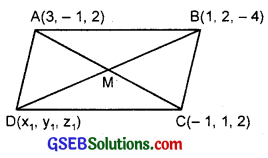Let the point D be (x1, y1, z1).
Now, (1, 0, 2) is the mid-point of diagonal BD also.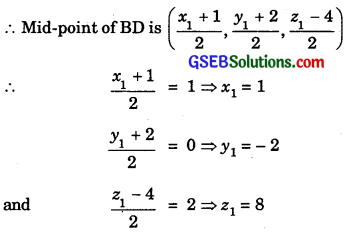∴ The coordinates of point D are (1, – 2, 8).Question 2.
Find the lengths of medians of the triangle whose vertices are A(0, 0, 6), B(0, 4, 0) and C(6, 0, 0).
Solution:
(i) The points B and C are (0, 4, 0) and (6, 0, 0).
∴ Middle point D of it is ($$\frac{0+6}{2}$$, $$\frac{4+0}{2}$$, $$\frac{0+0}{2}$$) or (3, 2, 0)
The point A is (0, 0, 6) and the point D is (3, 2, 0).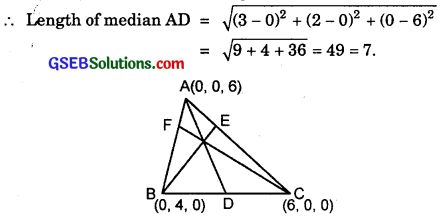(ii) The mid-point E of AC, where A is (0, 0, 6) and C is (6, 0, 0) is ($$\frac{0+6}{2}$$, $$\frac{0+0}{2}$$, $$\frac{6+0}{2}$$), i.e; (3, 0, 3).
Now, the point B is (0, 4, 0) and E is (3, 0, 3).
∴ Length of median BE = $$\sqrt{(3-0)^{2}+(0-4)^{2}+(3-0)^{2}}$$
= $$\sqrt{9+16+9}$$ = $$\sqrt{34}$$.

(iii) The mid-point F of the line segment joining the points A(0, 0, 6) and B(0, 4, 0) is
($$\frac{0+0}{2}$$, $$\frac{0+4}{2}$$, $$\frac{6+0}{2}$$) i.e; (0, 2, 3)
Now C is (6, 0, 0) and mid-point F of AB is (0, 2, 3).
∴ Length of median CF = $$\sqrt{(0-6)^{2}+(2-0)^{2}+(3-0)^{2}}$$
= $$\sqrt{36+4+9}$$ = 7.Question 3.
If the origin is the centroid of the triangle PQR with vertices P(2a, 2, 6), Q(- 4, 3b, – 10) and R(8, 14, 2c), then find the values of a, b and c.
Solution:
Vertices of ∆PQR are P(2a, 2, 6), Q(- 4, 3b, – 10) and R(8, 14, 2c).
∴ Centroid of the ∆ PQR isWe are given that centroid is origin i.e., (0, 0, 0).Question 4.
Find the coordinates of a point on y-axis which is at a distance of 5$$\sqrt{2}$$ from the point P(3, – 2, 5).
Solution:
A point lying on y-axis is A(0, y, 0).
The coordinates of point P are (3, – 2, 5).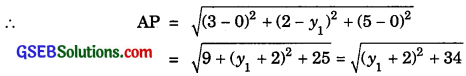But we have:
AP = 5$$\sqrt{2}$$
∴ $$\sqrt{\left(y_{1}+2\right)^{2}+34}$$ = 5$$\sqrt{2}$$
or (y1 + 2)2 = 50 – 34 = 16.
∴ y1 + 2 = ± 4.
∴ y1 = 2, – 6
Hence, there are two points (0, 2, 0) and (0, – 6, 0), which are at a distance of 5$$\sqrt{2}$$ from P(3, – 2, 5).Question 5.
A point R with x-coordinate 4 lies on the line segment joining the points P(2, – 3, 4) and Q(8, 0, 10). Find the coordinates of the point R.
Solution:
Let R divides PQ in the ratio k : 1, where P is (2, – 3, 4) and Q is (8, 0, 10).∴ The coordinates of point R are ($$\frac{8k+2}{k+1}$$, $$\frac{0-3}{k+1}$$, $$\frac{10k+4}{k+1}$$)
But x-coordinate of R is 4.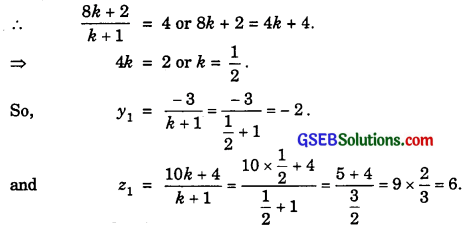∴ The point R is (4, – 2, 6).Question 6.
If A and B are the points (3, 4, 5) and (- 1, 3, – 7) respectively, find the equation of set of points P such that PA2 + PB2 = k2, where k is a constant.
Solution:
Let the coordinates of P be (x, y, z).
The given points are A(3, 4, 5) and B(- 1, 3, – 7).
So, PA2 + PB2 = [(x – 3)2 + (y – 4)2 + (z – 5)2] + [(x + 1)2 + (y – 3)2 + (z + 7)2]
= [x2 + y2 + z2 – 6x – 8y – 10z + 9 + 16 + 25] + [x2 + y2 + z2 + 2x – 6y + 14z + 1 + 9 + 49]
or 2(x2 + y2 + z2) – 4x – 14y + 4z + 50 + 59 = k2.
or 2(x2 + y2 + z2) – 4x – 14y + 4z + 109 – k2 = 0.